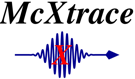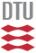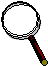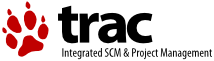McXtrace: Molecule_2state Component

# The `Molecule_2state` Component

Disordered optical-excitable molecule sample.

## Identification

• Author: Erik B Knudsen
• Origin: DTU Physics
• Date: October 2012
• Version: 1.0

## Description

```A sample model for pump probe experiments which models disordered molecules in a volume (rectangular,
cylindrical, or spherical). Molecules can be in one of two states (0 and 1).
Scattering is either specified through F vs. q scattering curves or as a set of atom positions from which
F vs. q is computed.
At t=-delta_t, a fraction of the molecules are put in state 1, from which they decay exponentially,
with time constant t_relax, into state 0. For t<-delta_t
all of the molecules are in the state specified by initial_state.
To improve statistics, scattering may be limited to a "forward" cone with opening angle in [psimin, psimax].
Furthermore, scattering may be restricted to the azimuthal segment between [etamin,etamax].

```

## Input parameters

Parameters in boldface are required; the others are optional.
Name Unit Description Default
form_factors File from which to read atomic form factors. Defualt amounts to use the one shipped with McXtrace. "FormFactors.txt"
state_0_file Isotropic scattering factors (parameterized by q), or atom positions are specified for state 0. NULL
state_1_file Isotropic scattering factors (parameterized by q), or atom positions are specified for state 1. NULL
nq Number of q-bins if F is to be computed from atom positions (Debye formalism). 512
material_datafile Where to read f1 and f2 factors from in order to handle absorption. "Be.txt"
delta_t s Delay between the exciting event t=0. delay is negative, i.e. delta_t>0 means the exciting event happens before t=0. 100e-9
excitation_yield Mean fraction of molecules that get excited. 0.2
t_relax s Mean relaxation time (into state 0) of excited molecules. 100e-9
initial_state Which state is Molecule_2state in for t 0
psimin rad Minimum scattering angle off the optical axis. 0
psimax rad Maximum scattering angle off the optical axis. M_PI_2
etamin rad Minimum scattering angle around the optical axis. -M_PI
etamax rad Maximum scattering angle around the optical axis. M_PI
yheight m Height of rectangular or cylindrical sample. 0
xwidth m Width of rectangular sample. 0
zdepth m Depth (thickness) of rectangular sample. 0
concentration m Concentration or packing factor of sample. 1
p_transmit m Fraction of statistics devoted to sample direct (unscattered) beam. 0.1
q_parametric 0: Assume that datafiles contains atom positions. 1: datafiles contains F vs. q data. 0
Emax Maximal energy for which scattering factors are computed. Must be larger than the maximal impinging energy. 80

## Output parameters

Name Unit Description Default
delta_q
prms
Prms
shape